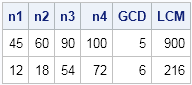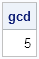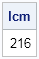My daughter's middle school math class recently reviewed how to compute the greatest common factor (GCF) and the least common multiple (LCM) of a set of integers. (The GCF is sometimes called the greatest common divisor, or GCD.) Both algorithms require factoring integers into a product of primes. While helping my daughter study, I wondered whether I could implement the GCF and LCM algorithms in SAS software.

It turns out that there are already two Base SAS functions that do the computations: the GCD Function computes the greatest common divisor for integer arguments, and the LCM function returns the least common multiple

You can call these functions in the SAS DATA step, as shown in the following example:

```data A; input n1 n2 n3 n4; GCD = gcd(n1,n2,n3,n4); LCM = lcm(n1,n2,n3,n4); datalines; 45 60 90 100 12 18 54 72 ;   proc print data=A nobs; run;```If you have an array of integers, you can also use the OF operator instead of using a comma-separated list.

In the SAS/IML language, it is sometimes inconvenient to call Base SAS functions that require lists of arguments. However, it occurred to me that the problem of computing the GCD of a vector of numbers can be accomplished by using an algorithm that always computes with exactly two integers at a time.

A simple example, illustrates the algorithm. Suppose you want to find the GCD of 24, 36, and 40. The GCD of 24 and 36 is 12. The GCD of 12 and 40 is 4. Therefore, the GCD of the three numbers is 4.

This example generalizes: to find the GCD of k natural numbers, first compute g as the GCD of the first k – 1 numbers, then find the GCD of g and the last element. By mathematical induction, you can compute the GCD of many numbers by computing pairwise GCDs. By using this pairwise-GCD algorithm, you can easily compute the GCD of elements in a SAS/IML vector by calling the Base SAS GCD function, as follows:

```proc iml; /* pairwise computation of the GCD of two or more natural numbers */ start GCDVec(x); gcd = gcd(x, x); do i = 3 to nrow(x)*ncol(x); gcd = gcd(gcd, x[i]); end; return( gcd ); finish;   x = {45, 60, 90, 100}; /* test it on four numbers */ gcd = GCDVec(x); print gcd;```In a similar way, you can compute the LCM of a SAS/IML vector by using the Base SAS LCM function and a pairwise algorithm:

```/* pairwise computation of the LCM of two or more natural numbers */ start LCMVec(x); lcm = lcm(x, x); do i = 3 to nrow(x)*ncol(x); lcm = lcm(lcm, x[i]); end; return( lcm ); finish;   x = {12, 18, 54, 72}; lcm = LCMVec(x); print lcm;```I like this algorithm because it leverages Base SAS functions to create a new SAS/IML function that operates on elements of a vector.

I can't think of any statistical methods that use the GCD or LCM, can you? If you know an application of the GCD or LCM in statistics, tell me about it by leaving a comment.

Share